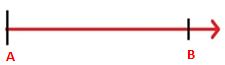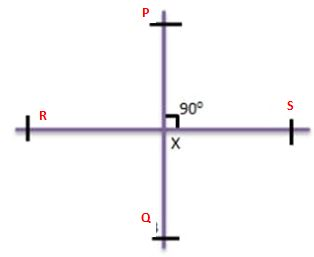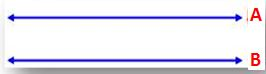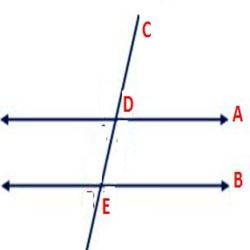# Lines and Angles – Definition, Types, Properties, Solved Problems

Lines and Angles concepts are explained by experts in order to help students to get excellent marks in their examination. The line is a combination of infinite points that has both directions infinitely. There are different lines available such as intersecting lines, perpendicular lines, transversal lines, etc. An angle is nothing but a combination of two rays with a common endpoint.

Based on the operations performed on a line, then the lines are classified as Parallel Lines, Perpendicular Lines, Transversal, etc. In the same way, the angles are classified as Complementary Angles, Supplementary Angles, Adjacent Angles, Vertically Opposite Angles based on their operation.

## Quick Links of Lines and Angles

Working on Lines and Angles problems will help students to get an idea about how to solve the problems. All the concept links are given below. Just check out the concepts and open the topic that is difficult for you. After that solve all the problems available on that topic to get a grip on that entire topic.

### Line Segment

The line segment is a line that has two endpoints with a fixed length.### Ray

A ray is a line that has only one endpoint and extends infinitely in another direction. From the below figure, point A is the and point.### Perpendicular Lines

Two lines are said to be Perpendicular Lines when they meet at a single point and forms a right angle. From the below figure, PQ and RS are two lines and they intersect at a point x. Also, the two lines PQ and RS form a right angle 90º.### Parallel Lines

Two lines are said to be Parallel Lines when they do not intersect each other and they have the same distance throughout the plane. From the below figure, A and B are two parallel lines.### Transversal Line

A transversal Line is a line that intersects two lines at distinct points. From the below figure, C is the Transversal Line, that intersects the lines A and B at two points D and E.### Properties of Lines

• The points those are not lie on the same line are called non-collinear points.
• The points that lie on the same line are called Collinear points

### Acute Angle

The angle that is less than the right angle is called Acute Angle.

### Obtuse Angle

The angle that is more than the right angle is called Acute Angle.

### Right Angle

The angle with 90 degrees is called as Right Angle.

### Straight Angle

The angle that is equal to 180 degrees is called Straight Angle.

### Complementary Angles

If the sum of the two angles is 90 degrees, then they are called Complementary Angles.

### Supplementary Angles

If the sum of the angles is 180 degrees, then those angles are called Supplementary Angles.

### Properties of Angles

• An angle is formed at a point where two rays intersecting.
• If the angle is more than 180 degrees and less than 360 degrees, then it is called a reflex angle.
• If the two angles are adjacent angles and add up to 180 degrees then they form linear pair.